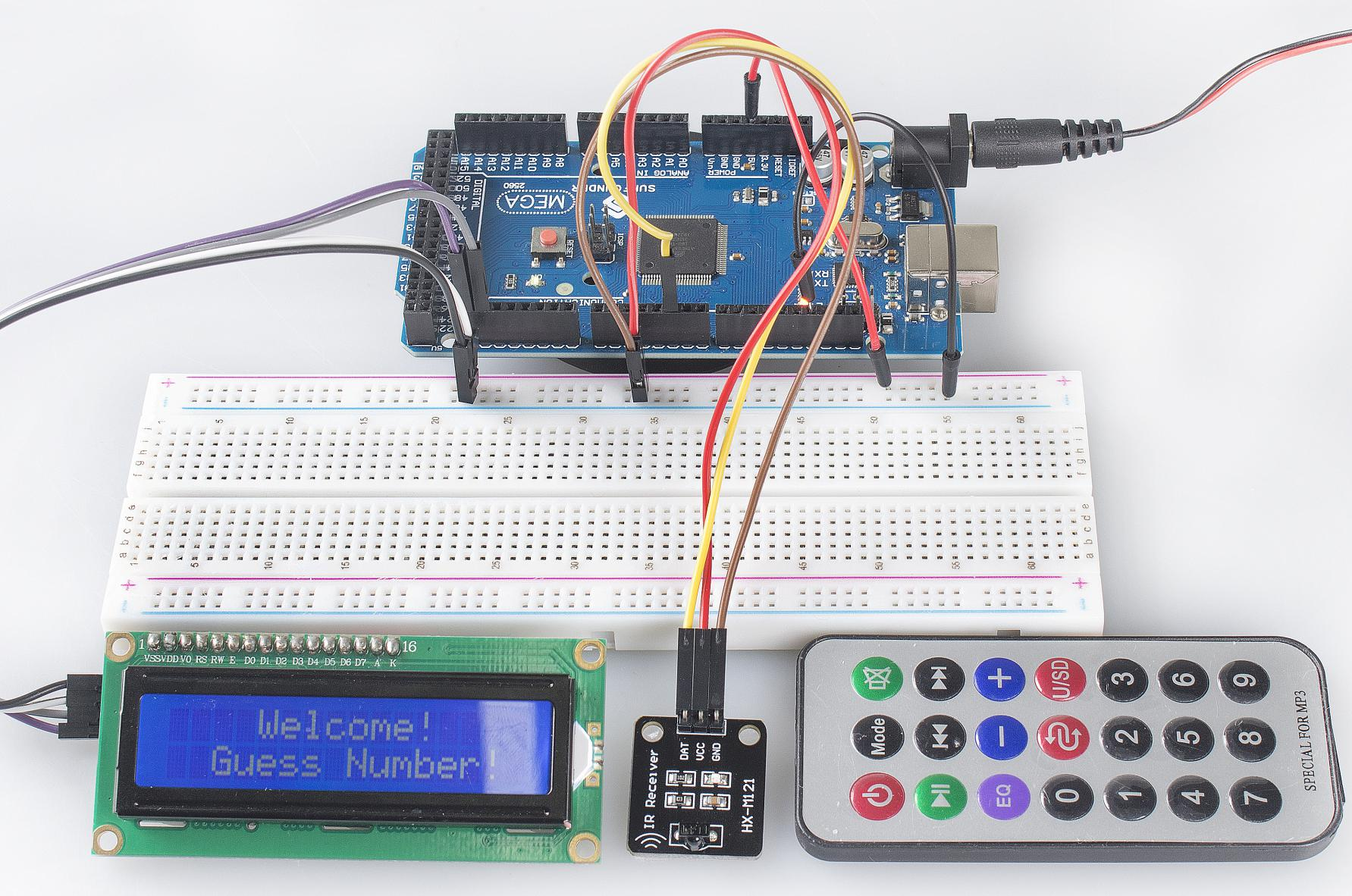3.4 Guess Number¶

Overview¶

Guessing Numbers is a fun party game where you and your friends take turns inputting a number (0~99). The range will be smaller with the inputting of the number till a player answers the riddle correctly. Then the player is defeated and punished. For example, if the lucky number is 51 which the players cannot see, and the player ① inputs 50, the prompt of number range changes to 50~99; if the player ② inputs 70, the range of number can be 50~70; if the player ③ inputs 51, he or she is the unlucky one. Here, we use IR Remote Controller to input numbers and use LCD to output outcomes.

Components Required¶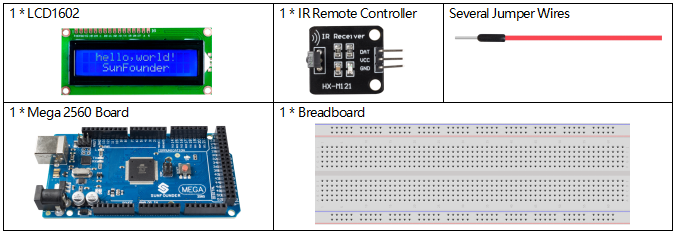Fritzing Circuit¶

In this example, the wiring of LCD1602 and infrared receiving module is as follows.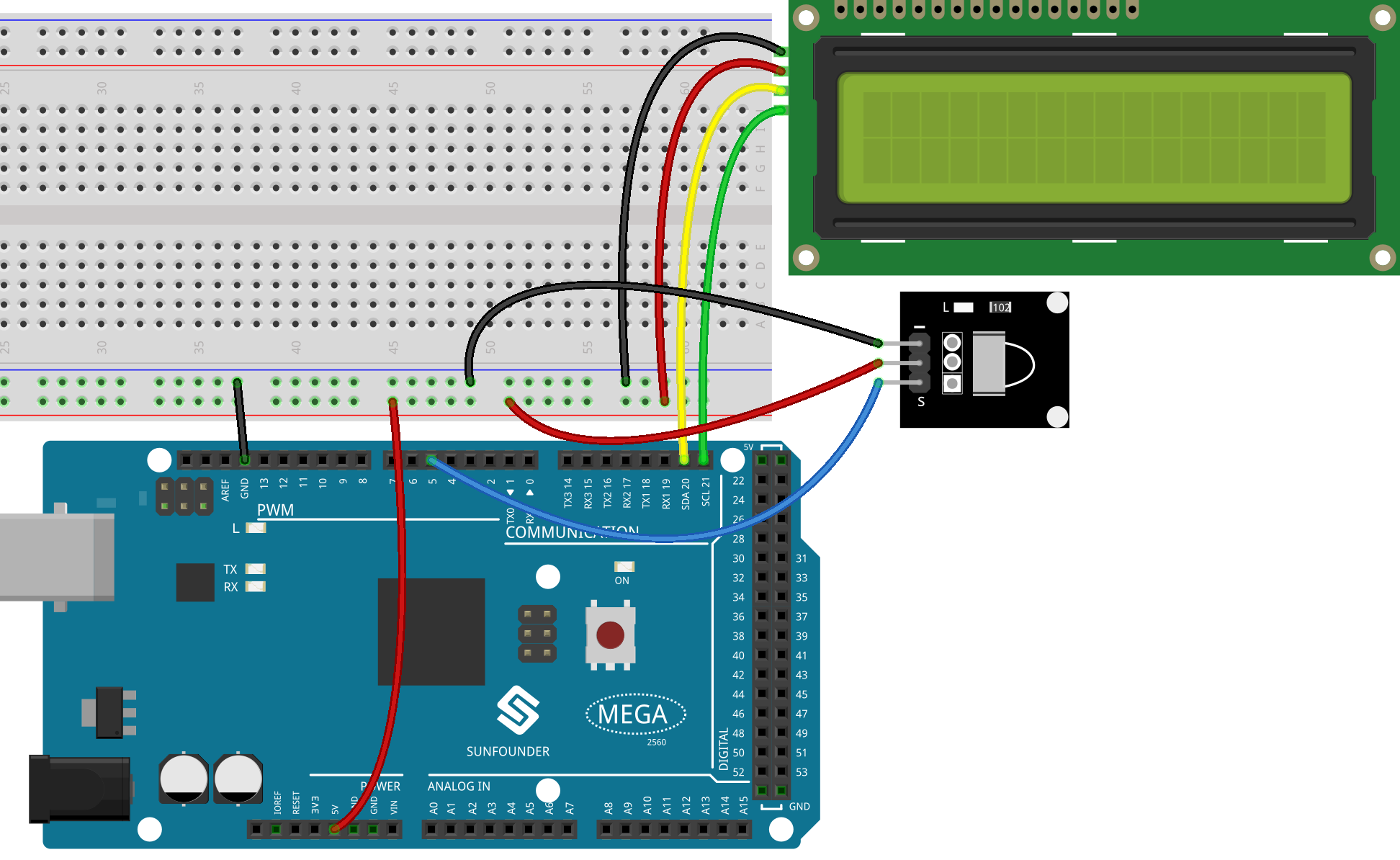Schematic Diagram¶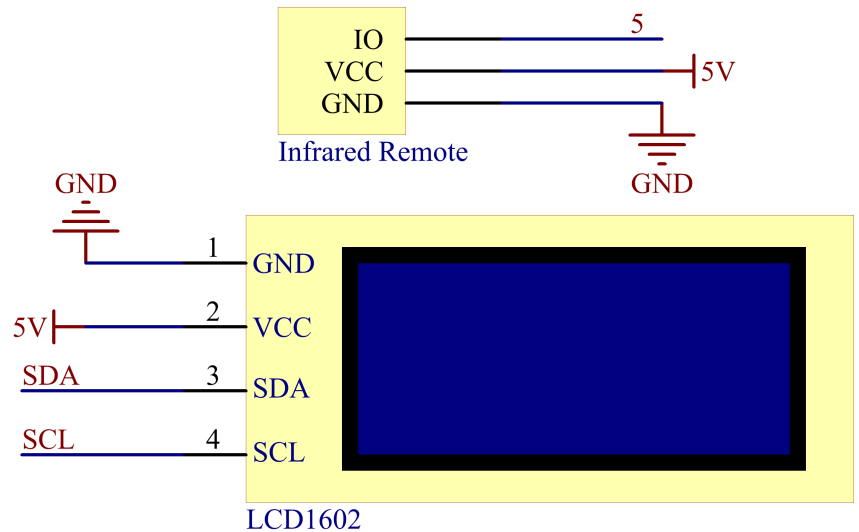Example Explanation¶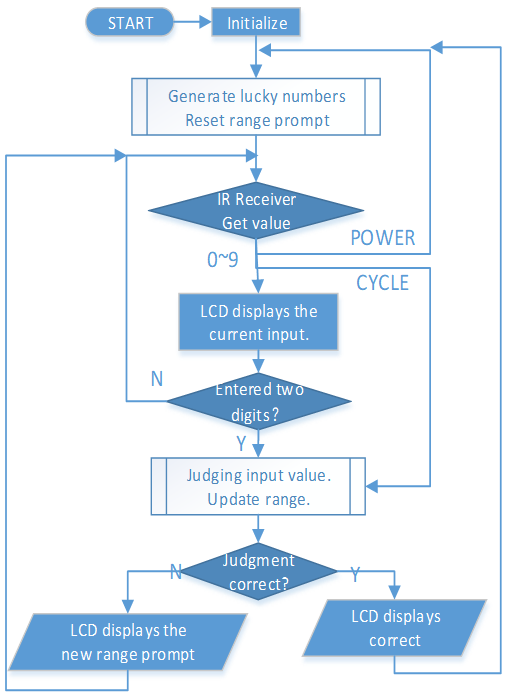In order to make the number guessing game become vivid and funny, we need to achieve the following functions:

1. The lucky number will be displayed when we start and reset the game, and the number range prompt is reset to 0 ~ 99.

2. LCD will display the number being input and the number range prompt.

3. After inputting two digits, there appears result judgment automatically.

4. If you input a single digit, you can press the CYCLE key (the key at the center of the Controller) to start the result judgment.

5. If the answer is not guessed, the new number range prompt will be displayed (if the lucky number is 51 and you enter 50, the number range prompt will change to 50~99).

6. The game is automatically reset after the lucky number is guessed, so that the player can play a new round.

7. The game can be reset by directly pressing the POWER button (the button in the upper left corner).

In conclusion, the work flow of the project is shown in the flow chart.

Phenomenon Picture¶